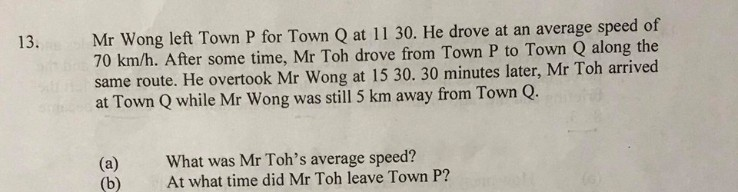# Questionhelp pls..

This is a distance speed time qestion.

1.  Wong. Start first. Speed = 70.

2. Toh. End first. 30 minute before wong, 5 km away.

 Time Speed Distance Wong 0.5 70 35 Toh 0.5 80 40

a)

0.5 × 70 = 35

35 + 5 = 40

40 ÷ 0.5 = 80 km/h

b)

2 × 70 = 140

140 + 40 = 180

180 ÷ 80 = 2.25 h = 2 h 15 minutes

2h 15 minute – 30 minute = 1 h 45 minutes

3 h 30 minutes – 1 h 45 minute = 1 h 45 minutes= 1.45 pm

😊

1 Reply 0 Likes

thank you very much…

0 Replies 0 Likes

From the overtook location,
Wong travelled = 0.5 X 70 = 35km,
That means Toh travelled in 30mins = 35 + 5km = 40km
a) Toh’s speed = 40/0.5 = 80km/h ###

Find the distance of Overtook location to Town P
Wong Took 4 hours,
Distance to Ovetook place = 70 X 4 = 280km
Time taken for Toh to reach 280km, = 280/80 = 3.5 hours
b) Time when Toh started his journey = 1530 – 3.5 hours = 12pm ###

1 Reply 0 Likes

thank you very much …

0 Replies 0 Likes